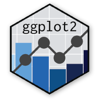Show Sidebar Hide Sidebar# geom_contour in ggplot2

How to make a contour in ggplot2 using geom_contour.

### New to Plotly?

Plotly's R library is free and open source!
You can set up Plotly to work in online or offline mode.
We also have a quick-reference cheatsheet (new!) to help you get started!

### Version Check

Version 4 of Plotly's R package is now available!
Check out this post for more information on breaking changes and new features available in this version.

library(plotly)
packageVersion('plotly')

##  '4.9.1'


### Basic geom_contour plot

geom_contour produces a similar output to geom_density_2d, except it uses a third variable for the values rather than frequency. The volcano dataset comes pre-loaded on R.

library(plotly)
library(reshape2)
df <- melt(volcano)

p <- ggplot(df, aes(Var1, Var2, z= value)) +
geom_contour() +
scale_fill_distiller(palette = "Spectral", direction = -1)
p <- ggplotly(p)

p


### Coloured Plot

See here for a list of colour palettes that come with the brewer (discrete) and distiller (continuous) packages.

library(plotly)
library(reshape2)
df <- melt(volcano)

p <- ggplot(df, aes(Var1, Var2, z= value, colour=stat(level))) +
geom_contour() +
scale_colour_distiller(palette = "YlGn", direction = 1)

ggplotly(p)


### Filled Plot

It's possible to colour in each of the layers, by changing geom_contour to stat_contour as below. As the edges of the graph indicate, filled contour plots only work when each layer is an enclosed shape rather than an open line; a geom more suited to this functionality would be geom_tile or geom_raster.

library(plotly)
library(reshape2)
df <- melt(volcano)

p <- ggplot(df, aes(Var1, Var2, z= value)) +
stat_contour(geom="polygon",aes(fill=stat(level))) +
scale_fill_distiller(palette = "Spectral", direction = -1)

ggplotly(p)